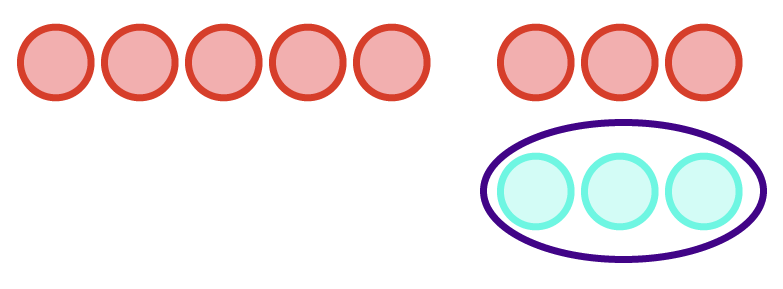## Notation and Modeling Subtraction of Integers

### Learning Outcomes

• Model subtraction of integers

You learn as a child how to subtract numbers through everyday experiences. For example, if you have 10 cheese crackers and eat 6 of them, you will have 4 cheese crackers left. Real-life experiences serve as models for subtracting positive numbers, but it is difficult to relate subtracting negative numbers to common life experiences. Most people do not have an intuitive understanding of subtraction when negative numbers are involved. Math teachers use several different models to explain subtracting negative numbers, but we will continue to use counters to model subtraction. Remember, the blue counters represent positive numbers and the red counters represent negative numbers.

Perhaps when you were younger, you read $5 - 3$ as five take away three. When we use counters, we can think of subtraction the same way. Through the next few examples, we will model four subtraction scenarios involving both positive and negative integers.

• $5 - 3$ ,   positive $-$ positive
• $- 5-\left(-3\right)$ ,   negative $-$ negative
• $-5 - 3$ ,   negative $-$ positive
• $5-\left(-3\right)$ ,   positive $-$ negative

### example

Model: $5 - 3$.

Solution:

 Interpret the expression. $5 - 3$ means $5$ take away $3$ . Model the first number. Start with $5$ positives.Take away the second number. So take away $3$ positives.Find the counters that are left.$5 - 3=2$ The difference between $5$ and $3$ is $2$ .

Now you can try a similar problem.

### try it

Model the expression:

$6 - 4$

Model the expression:

$7 - 4$

In the previous example we subtracted positive $3$ from positive $5$. Now we will subtract negative $3$ from negative $5$. Compare the results of this example to the previous example after you read through it.

### example

Model: $-5-\left(-3\right)$

You can try a similar problem.

### try it

Model the expression:

$-6-\left(-4\right)$

Model the expression:

$-7-\left(-4\right)$

Notice that the previous two examples are very much alike.

• First, we subtracted $3$ positives from $5$ positives to get $2$ positives.
• Then we subtracted $3$ negatives from $5$ negatives to get $2$ negatives.

Each example used counters of only one color, and the “take away” model of subtraction was easy to apply.Now let’s see what happens when we subtract one positive and one negative number. We will need to use both positive and negative counters and sometimes some neutral pairs, too. Remember that adding a neutral pair does not change the value (it’s like adding zero to any number).

### example

Model: $-5 - 3$

Solution:

 Interpret the expression. $-5 - 3$ means $-5$ take away $3$ . Model the first number. Start with $5$ negatives.Take away the second number. So we need to take away $3$ positives. But there are no positives to take away. Add neutral pairs until you have $3$ positives.Now take away $3$ positives.Count the number of counters that are left.$-5 - 3=-8$ The difference of $-5$ and $3$ is $-8$ .

When modeling these types of problems, you will always need to add neutral pairs to the initial value until you have enough of the correct type of counters to remove.

Now you can try a similar problem.

### try it

Model the expression:

$-6 - 4$

Model the expression:

$-7 - 4$

Now we will subtract a negative number from a positive number. Think of this as taking away the negative.

### example

Model: $5-\left(-3\right)$

Now you can try a similar problem.

### try it

Model the expression:

$6-\left(-4\right)$

Model the expression:
$7-\left(-4\right)$

Now we will do an example that summarizes the situations above, with different numbers. Recall the different scenarios:

• subtracting a positive number from a positive number
• subtracting a negative number from a negative number
• subtracting a positive number from a negative number
• subtracting a negative number from a positive number

### example

Model each subtraction.

1. $8 − 2$
2. $−8 − (−3)$
3. $−5 − 4$
4. $6 − (−6)$

Now you can try a similar problem.

### try it

Model each subtraction.

1. $7 - (-8)$
2. $-7 - (-2)$
3. $4 - 1$
4. $-6 - 8$

Model each subtraction.

1. $4 - (-6)$
2. $-8 - (-1)$
3. $7 - 3$
4. $-4 - 2$

Each of the examples so far have been carefully constructed so that the sign of the answer matched the sign of the first number in the expression.  For example, in  $−5 − 4$, the result is $-9$, which is the same sign as $-5$. Now we will see subtraction where the sign of the result is different from the starting number.

### example

Model each subtraction expression:

1. $2 - 8$
2. $-3-\left(-8\right)$

Now you can try a similar problem.

### try it

Model each subtraction expression.

1. $7 - 9$
2. $-5-9$

Model each subtraction expression.

1. $5 - 8$
2. $-7-\left(-10\right)$

When you subtract two integers, there are two possibilities, either the result will have a different sign from the starting number, or it will have the same sign.

Watch the video below to see more examples of modeling integer subtraction with color counters.

## Contribute!

Did you have an idea for improving this content? We’d love your input.